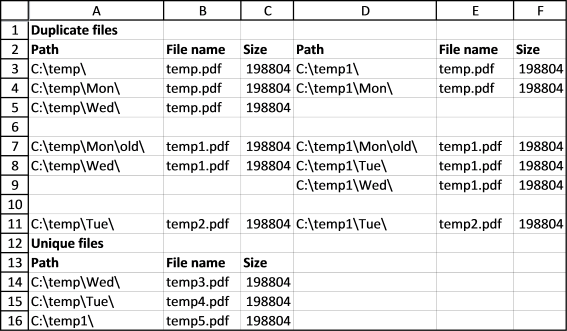Author: Oscar Cronquist Article last updated on December 26, 2018

Today I would like to share a macro that compares the content in two different folders and their sub folders. It compares the file names but not the file sizes.

In this example the picture shows the content in these two folders:

• c:\temp\
• c:\temp1\The file information for duplicate files in the first folder are in columns A to C, the second folder are in columns D to F.

As you can see, duplicate file names are together. Duplicate file names in the same folder tree and the corresponding folder tree are grouped together.

Unique files from both folders are in columns A to C, below the duplicate file list.

If you have folders with a lot of files and sub folders the macro may take a while to finish.

What happens when you start the macro?

1. Select the first folder
2. Select the second folder
3. A new sheet is inserted
4. The file data is saved to the new sheet
5. Macro ends

Where do I save the code?

1. Copy the vba code below
2. Go to the VB Editor (Alt + F11)
3. Click "Insert" on the menu
4. Click "Module"
5. Paste code to code window

VBA Code

Sub CompareContentsofTwoFolders()
Dim pth1 As String, pth2 As String
Dim r1 As Single, r2 As Single
Dim arrd() As Variant
Dim arru() As Variant
ReDim arrd(0 To 5, 0)
ReDim arru(0 To 2, 0)

With Application.FileDialog(msoFileDialogFolderPicker)
.Show
pth1 = .SelectedItems(1) & "\"
End With

With Application.FileDialog(msoFileDialogFolderPicker)
.Show
pth2 = .SelectedItems(1) & "\"
End With

Set x = ActiveSheet

Application.ScreenUpdating = False
x.Range("A1") = "Duplicate files"
x.Range("A2") = "Path"
x.Range("B2") = "File name"
x.Range("C2") = "Size"
x.Range("D2") = "Path"
x.Range("E2") = "File name"
x.Range("F2") = "Size"
x.Range("A:F").Font.Bold = False
x.Range("A1:F2").Font.Bold = True

Recursive pth1

Lrow = ActiveSheet.Range("A" & Rows.Count).End(xlUp).Row
x.Range("A2:C" & Lrow).Sort Key1:=x.Range("B1"), Header:=xlYes

arr1 = x.Range("A3:C" & Lrow).Value

x.Range("A3:C" & Lrow).Clear

Recursive pth2

Lrow = ActiveSheet.Range("A" & Rows.Count).End(xlUp).Row
x.Range("A2:C" & Lrow).Sort Key1:=x.Range("B1"), Header:=xlYes

arr2 = x.Range("A3:C" & Lrow).Value

x.Range("A3:C" & Lrow).Clear

For r1 = LBound(arr1, 1) To UBound(arr1, 1)
chk = False

If r1 > 1 Then

If arr1(r1, 2) = arr1(r1 - 1, 2) Then

For r3 = UBound(arrd, 2) To LBound(arrd, 2) Step -1
If arrd(2, r3) <> "" And arrd(1, r3) <> arr1(r1, 2) Then Exit For
If arrd(1, r3) = arr1(r1, 2) Then
If r3 = UBound(arrd, 2) Then ReDim Preserve arrd(UBound(arrd, 1), UBound(arrd, 2) + 1)
arrd(0, r3 + 1) = arr1(r1, 1)
arrd(1, r3 + 1) = arr1(r1, 2)
arrd(2, r3 + 1) = arr1(r1, 3)
ReDim Preserve arrd(UBound(arrd, 1), UBound(arrd, 2) + 1)
Exit For
End If
Next r3

For r3 = UBound(arru, 2) To LBound(arru, 2) Step -1
If arru(2, r3) <> "" And arru(1, r3) <> arr1(r1, 2) Then Exit For
If arru(1, r3) = arr1(r1, 2) Then
If r3 = UBound(arru, 2) Then ReDim Preserve arru(UBound(arru, 1), UBound(arru, 2) + 1)
arru(0, r3 + 1) = arr1(r1, 1)
arru(1, r3 + 1) = arr1(r1, 2)
arru(2, r3 + 1) = arr1(r1, 3)
ReDim Preserve arru(UBound(arru, 1), UBound(arru, 2) + 1)
Exit For
End If
Next r3
GoTo jmp
End If
End If

For r2 = LBound(arr2, 1) To UBound(arr2, 1)

If arr2(r2, 2) = arr1(r1, 2) Then

If chk = False Then
arrd(0, UBound(arrd, 2)) = arr1(r1, 1)
arrd(1, UBound(arrd, 2)) = arr1(r1, 2)
arrd(2, UBound(arrd, 2)) = arr1(r1, 3)

Else
arrd(0, UBound(arrd, 2)) = ""
arrd(1, UBound(arrd, 2)) = ""
arrd(2, UBound(arrd, 2)) = ""
End If

arrd(3, UBound(arrd, 2)) = arr2(r2, 1)
arrd(4, UBound(arrd, 2)) = arr2(r2, 2)
arrd(5, UBound(arrd, 2)) = arr2(r2, 3)

arr2(r2, 1) = ""

ReDim Preserve arrd(UBound(arrd, 1), UBound(arrd, 2) + 1)
chk = True

End If

Next r2

If chk = False Then
arru(0, UBound(arru, 2)) = arr1(r1, 1)
arru(1, UBound(arru, 2)) = arr1(r1, 2)
arru(2, UBound(arru, 2)) = arr1(r1, 3)
ReDim Preserve arru(UBound(arru, 1), UBound(arru, 2) + 1)
End If
jmp:
Next r1

For r2 = LBound(arr2, 1) To UBound(arr2, 1)
If arr2(r2, 1) <> "" Then
arru(0, UBound(arru, 2)) = arr2(r2, 1)
arru(1, UBound(arru, 2)) = arr2(r2, 2)
arru(2, UBound(arru, 2)) = arr2(r2, 3)
ReDim Preserve arru(UBound(arru, 1), UBound(arru, 2) + 1)
End If
Next r2

x.Range("A3").Resize(UBound(arrd, 2) + 1, UBound(arrd, 1) + 1) = Application.Transpose(arrd)

x.Range("A" & UBound(arrd, 2) + 3) = "Unique files"
x.Range("A" & UBound(arrd, 2) + 4) = "Path"
x.Range("B" & UBound(arrd, 2) + 4) = "File name"
x.Range("C" & UBound(arrd, 2) + 4) = "Size"
x.Range("A" & UBound(arrd, 2) + 3 & ":C" & UBound(arrd, 2) + 4).Font.Bold = True
x.Range("A" & UBound(arrd, 2) + 5).Resize(UBound(arru, 2) + 1, UBound(arru, 1) + 1) = Application.Transpose(arru)

x.Columns("A:F").AutoFit

Application.ScreenUpdating = True
End Sub

Sub Recursive(FolderPath As String)
Dim Value As String, Folders() As String
Dim Folder As Variant, a As Long
ReDim Folders(0)
If Right(FolderPath, 2) = "\\" Then Exit Sub
Value = Dir(FolderPath, &H1F)
Do Until Value = ""
If Value = "." Or Value = ".." Then
Else
If GetAttr(FolderPath & Value) = 16 Or GetAttr(FolderPath & Value) = 48 Then
Folders(UBound(Folders)) = Value
ReDim Preserve Folders(UBound(Folders) + 1)
Else
Lrow = ActiveSheet.Range("A" & Rows.Count).End(xlUp).Row + 1
ActiveSheet.Range("A" & Lrow) = FolderPath
ActiveSheet.Range("B" & Lrow) = Value
ActiveSheet.Range("C" & Lrow) = FileLen(FolderPath & Value)
End If
End If
Value = Dir
Loop
For Each Folder In Folders
Recursive FolderPath & Folder & "\"
Next Folder
End Sub